# Differential Equation

FIRST ORDER DIFFERENTIAL EQUATION
Basic Concepts and Ideas

Differential Equation: – An equation involving one dependent variable and its derivatives with respect to one or more independent variable is called as differential equation.
Example.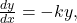,Ordinary differential equation: – An ordinary differential equation is one in which there is only one independent variable, so that all the derivatives occurring in it are ordinary derivatives.
Ex.Partial differential equation: – A Partial differential equation is one involving more than one independent variable, so that all the derivatives occurring in it are partial derivatives.
Example.Order of differential equation: – The order of the differential equation is the order of the highest derivatives present.
Example.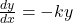(First order)(First order)(Second order)

Degree of differential equation: – The degree of differential equation is the exponent of highest order derivatives present in a differential equation after the differential equation has been rationalised and made free of fractional powers with regards to all derivatives present.
Ex.(Degree 1)(Degree 2)(Degree 1)
First order differential equation: -An equation contains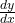,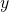and function of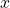is called as first order differential equation.
Ex.Solution of differential equation: – Any function free from derivatives, satisfying the differential equation identically is said to be a solution of differential equation.
Example.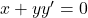has a solution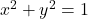Differentiating with respect to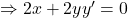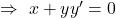which is a solution of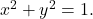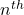order ordinary differential equation: –
Anorder differential equation in general form is

(1)Solution of (1) containing arbitrary constant is called as general solution.
Any solution obtained from the general solution by giving a particular value to the arbitrary constants is known as particular solution.

Initial Value problem: – A differential equation together with an initial condition is known as initial value problem, which can be expressed in the form of: –It means at x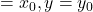Singular solution: -A differential equation may sometimes have an additional solution that cannot be obtained from the general solution and is then called a singular solution.

1.3 SEPARABLE DIFFERENTIAL EQUATION

Take the first order differential equation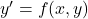(1)
If Equation (1) can be reduced in the form of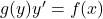(i.e. if we can separate the variables in x and y) Then its solution of equation (1) is